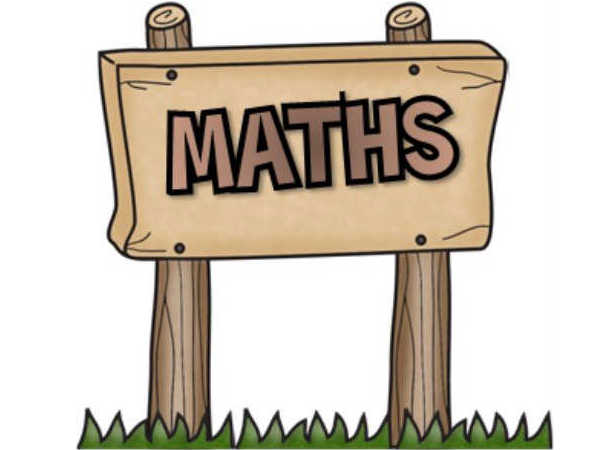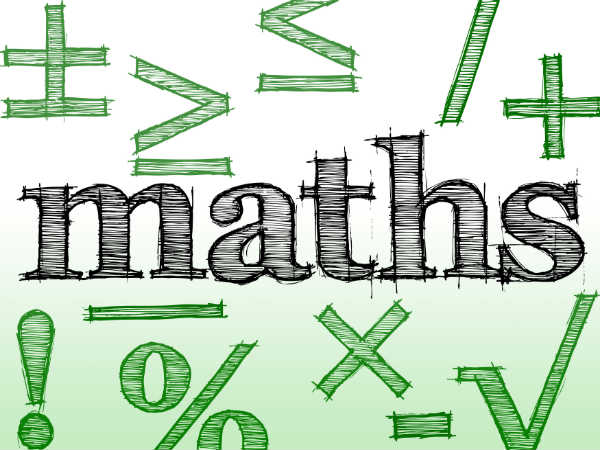CBSE Class 10 Mathematics 2018 Important Topics, Solved Answers And Model Question Papers

By Malavikka

Maths is a subject that could get you a straight zero or 100. Scoring well in the class 10 maths board exams will not only give you a strong foundation to the concepts but will also give you a lifelong recognition of your ability in maths.

CBSE class 10 mathematics exam is scheduled on 28 March 2018. There is hardly more than a month left for the students to finish their preparations in order to ace the exam. CareerIndia presents to you CBSE class 10 mathematics 2018 important topics, solved questions and model question papers that are important for you to study now.How To Score More Than 90 In Maths For CBSE Class 12 Board Exam?

CBSE Class 10 Mathematics Syllabus

 UNIT NUMBER UNIT NAME 1 Number Systems 2 Algebra 3 Co-ordinate Geometry 4 Geometry 5 Trignometry 6 Mensuration 7 Statistics and ProbabilityTop 10 Colleges In India For Maths In 2018

First, let's look at the chapter-wise important topics

 Chapter Number Topic 1 Real Numbers 2 Polynomials 3 Linear Equations in Two Variables 4 Quadratic Equations 5 Arithmetic Progressions 6 Triangles 7 Coordinate Geometry 8 Introduction to Trigonometry 9 Some Applications of Trigonometry 10 Circles 11 Constructions 12 Areas Related to Circles 13 Surface Areas and Volume 14 Statistics 15 ProbabilityIIT Madras Offers Free Online Mathematics Course 2018 In Collaboration With AICTE And MHRD

Real Numbers

Question

Use Euclid's Algorithm to find the HCF of 135 and 225

Solution

225>135

Use the division lemma to divide the two positive numbers:

225=135 + 1x90

135=90+45

90=2x45

225=135 + 2x45

Since 45 divides 225 and 135 exactly, 45 is the HCFMaths Aptitude Questions Types For Entrance Exams and Recruitment Preparation

Polynomials

Question

If a and b are the zeroes of the quadratic polynomial f(x) = 6x^2 + x = 2, find the value of a/b + b/a

Solution

f(x) = 6x^2 + x = 2

Since a and b are the zeroes of the given polynomial,

Sum of zeroes (a+b) = -1/6

ab = -1/3

a/b + b/a = ((a+b)^2 - 2ab)/ab7 Mathematics Shortcuts That Can Blow You Down

Triangles

Find out if the following statements are true or false:

Subscribe Now

--Or--
Select a Field of Study
Select a Course
Select UPSC Exam
Select IBPS Exam
Select Entrance Exam